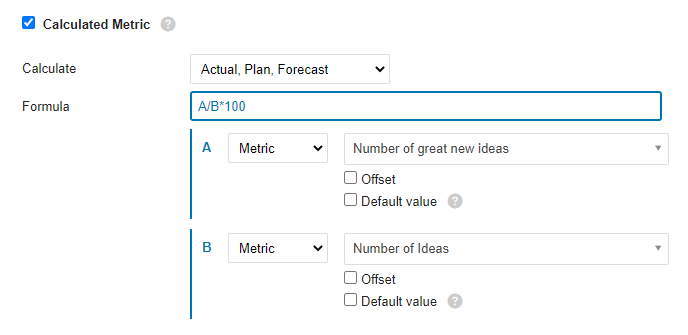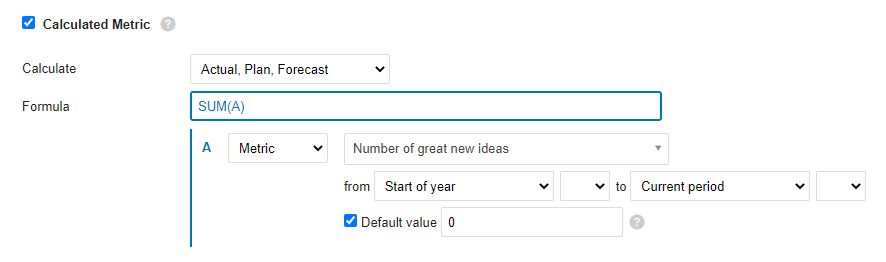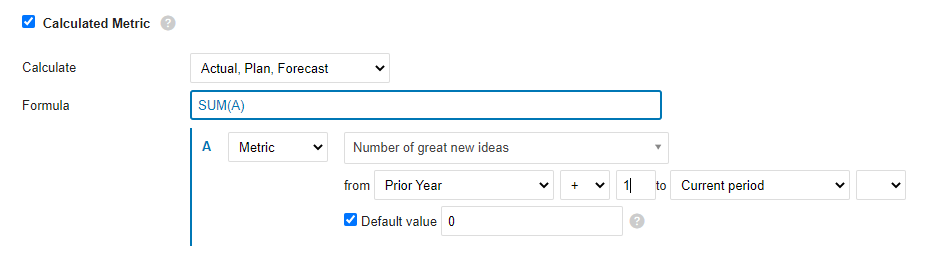Support Center / Metric Dashboards / Calculated Metrics & Advanced Formulas

# Calculated Metrics & Advanced Formulas

### Ratio (Percentage): A/B*100

Divide metric A by metric B and multiply by 100 to get a percentage.### YTD

YTD value is calculated as a sum of all values from the beginning of the year to the current period. If there are missing values for any number of periods, you can use the Default value option to fill the gaps and ensure, that the formula will be calculated.### Rolling YTD

Similarly as YTD, sum all the values from the prior-year period (+1, we want to start from the next period in the prior year)  to the current period.## LIST OF FUNCTIONS

### ABS

Returns the absolute value of a number. The absolute value of a number is the same unsigned number.

ABS (number)

The syntax of the ABS function contains the following arguments:

• Number: Mandatory argument. The real number whose absolute value you want to find out.

### LOG10

Returns the decimal logarithm of a number.

LOG (number)

The syntax of the LOG function has the following arguments:

• Number: Mandatory argument. This is a positive real number whose decimal logarithm you want to calculate.

### MOD

Returns the signed remainder of a division, after one number is divided by another.

MOD (dividend, divisor)

The syntax of the MOD function contains the following arguments:

• Dividend: Mandatory argument. Dividend is the number divided by divisor
• Divisor: Mandatory argument. This is the number by which the dividend is divided.

### POWER

Returns the power of a number.

POWER (number, power)

The syntax of the POWER function contains the following arguments:

• Number: Mandatory argument. This is the basis for exponentiation. It can be any real number.
• Power: Mandatory argument. This is the exponent by which the base is to be amplified.

### ROUND

Rounds a number to the specified number of digits.

ROUND (number, digits)

The syntax of the ROUND function contains the following arguments.

• Number: Mandatory argument. This is the number you want to round.
• Digits: Required argument. Specifies the number of digits to which you want to round the number.

### ROUNDUP

Rounds a number up, from zero.

ROUNDUP (number, digits)

The syntax of the ROUNDUP function contains the following arguments:

• Number: Mandatory argument. This is any real number you want to round up.
• Digits: Required argument. Specifies how many decimal places the number should be rounded.

### ROUNDDOWN

Rounds a number down to zero.

ROUNDDOWN (number, digits)

The syntax of the ROUNDDOWN function has the following arguments:

• Number: Mandatory argument. This is any real number you want to round down.
• Digits: Required argument. Specifies how many decimal places the number should be rounded.

### SQRT

Returns the square root of a given number.

SQRT (number)

The syntax of the SQRT function contains the following arguments:

• Number: Mandatory argument. This is the number whose square root you want to know.

### TRUNC

Shortens a number to an integer by removing the decimal or fractional part of the number.

TRUNC (number, [digits])

The syntax of the TRUNC function has the following arguments:

• Number: Mandatory argument. This is the number you want to shorten.
• Decimals: Optional argument. This is the number of decimal places in the abbreviated number. The default setting for tenths is 0 (zero).

### AND

Returns TRUE if all arguments are TRUE.

AND (A, B)

The syntax of the AND function has the following arguments:

• A: Mandatory argument. Condition
• B: Mandatory argument. Condition

### STEED

Returns TRUE if at least one argument is TRUE.

OR (A, B)

The syntax of the OR function has the following arguments:

• A: Mandatory argument. Condition
• B: Mandatory argument. Condition

### XOR

The XOR function returns a logical exclusive or all arguments.

XOR (A, B)

The syntax of the XOR function has the following arguments:

• A: Mandatory argument. Condition
• B: Mandatory argument. Condition

### NOT

Performs a logical negation of a function argument.

NOT(A)

The syntax of the NOT function has the following arguments:

• A: Mandatory argument. Condition

TRUE
Returns the logical value TRUE.

FALSE
Returns the logical value FALSE.

### IF

One of the most popular functions in TeamGuru is the IF function, which allows you to logically compare a value with expectations.

An IF statement can have two results. The first result is returned if the condition evaluates as true. Otherwise, the second result is returned.

IF (condition, a, b)

The syntax of the IF function has the following arguments:

• condition: Required argument. Condition for evaluation
• a: Mandatory argument. The result of the IF function if the condition is TRUE
• b: Mandatory argument. The result of the IF function if the condition is FALSE

### IFERROR

If the formula evaluates to an error, it returns the specified value. Otherwise, it returns the result of the formula.

IFERROR (value, value_on_error)

The syntax of the IFERROR function has the following arguments:

• value: Required argument. This is the argument in which the error is tested.
• value_on_error: Required argument. The value that is returned if the formula results in an error.

### ISNULL

If the result of the formula is an empty value, it returns TRUE

ISNULL (value)

The syntax of the ISNULL function has the following arguments:

• value: Required argument. This is an argument that tests whether it is an empty or null value

### AVERAGE

Calculates the average (arithmetic mean) of values.

AVERAGE (list_of_values)

The syntax of the AVERAGE function has the following arguments:

• value_list: Required argument. A list of values ​​in TeamGuru defined as a range of periods.

### SUM

Calculates the sum of all values.

SUM (list_values)

The syntax of the SUM function has the following arguments:

• value_list: Required argument. A list of values ​​in TeamGuru defined as a range of periods.

### COUNT

Returns the number of values ​​in a list.

COUNT (values_list)

The syntax for the COUNT function has the following

arguments:

• values_list: Required argument. A list of values ​​in TeamGuru defined as a range of periods.

### MAX

Returns the maximum value from a list of values.

MAX (values_list)

The syntax of the MAX function has the following arguments:

• values_list: Required argument. A list of values ​​in TeamGuru defined as a range of periods.

### MIN

Returns the minimum value from a list of values.

MIN (values_list)

The syntax of the MIN function has the following arguments:

• values_list: Required argument. A list of values ​​in TeamGuru defined as a range of periods.

### MEDIAN

Returns the median of the entered numbers. A median is a number that lies in the middle of a set of numbers.

MEDIAN (values_list)

The syntax of the MEDIAN function has the following arguments:

• values_list: Required argument. A list of values ​​in TeamGuru defined as a range of periods.

### STDEVP

Returns the standard deviation of a base file determined from a random selection. The standard deviation expresses how the values ​​differ from the mean value (mean value).

STDEVP (values_list)

The syntax of the STDEVP function has the following arguments:

• values_list: Required argument. A list of values ​​in TeamGuru defined as a range of periods.

### STDEVA

Calculates the standard deviation of a selection. The standard deviation is the degree of variance of the values ​​from the mean value.

STDEVA (values_list)

The syntax of the STDEVA function has the following arguments:

• values_list: Required argument. A list of values ​​in TeamGuru defined as a range of periods.

### LAST

Returns the last value entered from the list of values. Ignores empty and null values.

LAST (values_list)

The syntax of the LAST function has the following arguments:

• values_list: Required argument. A list of values ​​in TeamGuru defined as a range of periods.